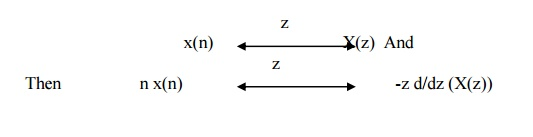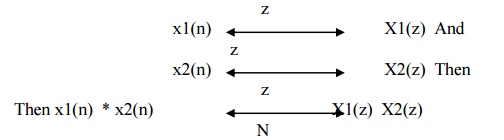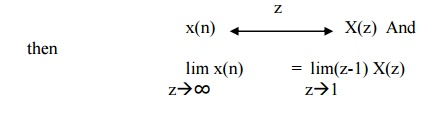Home | Plot and Properties of Z Transform

# Plot and Properties of Z Transform

Z Transform Plot - Fig show the plot of z transforms. The z transform has real and imaginary parts. Thus a plot of imaginary part versus real part is called complex z-plane. The radius of circle is 1 called as unit circle.

Z TRANSFORM PLOTFig show the plot of z transforms. The z transform has real and imaginary parts. Thus a plot of imaginary part versus real part is called complex z-plane. The radius of circle is 1 called as unit circle.

This complex z plane is used to show ROC, poles and zeros. Complex variable z is also expressed in polar form as Z= rejω where r is radius of circle is given by |z| and ω is the frequency of the sequence in radians and given by z.Tutorial problems:

Q) Determine z transform of following signals. Also draw ROC.

i)     x(n) = {1,2,3,4,5}

ii)    x(n)={1,2,3,4,5,0,7}

Q) Determine z transform and ROC for x(n) = (-1/3)n u(n) –(1/2)n u(-n-1).

Q) Determine z transform and ROC for x(n) = [ 3.(4n)–4(2n)] u(n).

Q) Determine z transform and ROC for x(n) = (1/2)n u(-n).

Q) Determine z transform and ROC for x(n) = (1/2)n {u(n) – u(n-10)}.

Q) Find linear convolution using z transform. X(n)={1,2,3} & h(n)={1,2}

PROPERTIES OF Z TRANSFORM (ZT)

1) Linearity

The linearity property states that ifz Transform of linear combination of two or more signals is equal to the same linear combination of z transform of individual signals.

2) Time shifting

The Time shifting property state that ifThus shifting the sequence circularly by ‘k‘ samples is equivalent to multiplying its z transform by z –k

3) Scaling in z domainThus scaling in z transform is equivalent to multiplying by an  in time domain.

4) Time reversal PropertyIt means that if the sequence is folded it is equivalent to replacing z by z-1 in z domain.

5) Differentiation in z domain

The Differentiation property states that if6) Convolution Theorem

The Circular property states that ifConvolution of two sequences in time domain corresponds to multiplication of its Z transform sequence in frequency domain.

7) Correlation Property

The Correlation of two sequences states that if8) Initial value Theorem

Initial value theorem states that if9) Final value Theorem

Final value theorem states that ifStudy Material, Lecturing Notes, Assignment, Reference, Wiki description explanation, brief detail
Discrete Time Systems and Signal Processing : Discrete Time System Analysis : Plot and Properties of Z Transform |